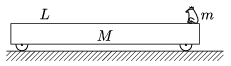Mathematical and Physical Journal
for High Schools
Issued by the MATFUND Foundation
 Already signed up? New to KöMaL?

#Problem P. 4120. (December 2008)

P. 4120. An easily moveable trolley of mass M and of length L is at rest on the horizontal tabletop. At the end of the trolley there is a frog of mass m. What must the initial speed of the frog be at which it has to leap in the direction ofmeasured from the horizontal in order to land exactly at the other end of the trolley? (Data: M=0.6 kg, m=54 g, L=81 cm,=45o.)(5 pont)

Deadline expired on January 12, 2009.

### Statistics:

 163 students sent a solution. 5 points: 110 students. 4 points: 17 students. 3 points: 13 students. 2 points: 5 students. 1 point: 3 students. Unfair, not evaluated: 15 solutionss.

Problems in Physics of KöMaL, December 2008